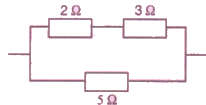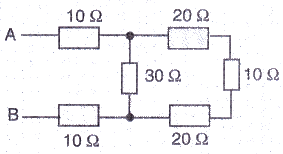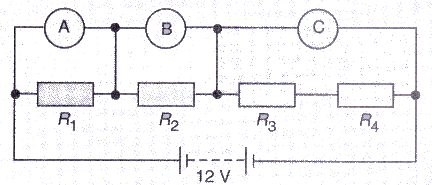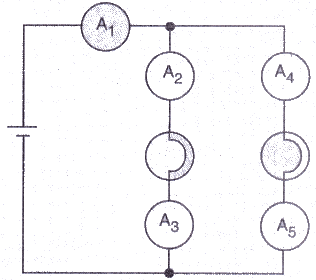Courses

# Solutions of Electricity (Page No- 44) - Physics By Lakhmir Singh, Class 10 Class 10 Notes | EduRev

## Class 10 : Solutions of Electricity (Page No- 44) - Physics By Lakhmir Singh, Class 10 Class 10 Notes | EduRev

The document Solutions of Electricity (Page No- 44) - Physics By Lakhmir Singh, Class 10 Class 10 Notes | EduRev is a part of the Class 10 Course Class 10 Physics Solutions By Lakhmir Singh & Manjit Kaur.
All you need of Class 10 at this link: Class 10

Lakhmir Singh Physics Class 10 Solutions Page No:44

Question 47:
How will you connect three resistors of 2 Ω, 3 Ω and 5 Ω respectively so as to obtain a resultant resistance of
2.5 Ω ? Draw the diagram to show the arrangement.

Solution :

Given: Three resistances of 2ohms. 3ohms. 5ohms.

Their resultant. R = 2.5ohmsResistance of first line = 2+3 = 5 ohm

So, 1/R = 1/5 + 1/5

On solving we get that

R = 2.5ohms

Question 48:
How will you connect three resistors of resistances 2 Ω, 3 Ω and 6Ω obtain a total n si stance of: (a) 4 Ω., and (b) 1Ω?

Solution :

(a) Connect 2ohms resistor in series with a parallel combination of 3ohms and 6ohms.
(b) Connect 2ohms. 3ohms. and 6ohms in parallel.

Question 49:
What is (a) highest, and (b) lowest, resistance which can be obtained by combining Com resistors having the following resistances ?
4 Ω, 8 Ω, 12 Ω, 24 Ω

Solution :

(a) For obtaining the highest resistance by combining the given resistances, we must connect them in series.

We get.

R = 4+8+12+24 = 48ohms

(b) For obtaining the lowest resistance by combining the given resistances, we must connect them in parallel.

We get.

1/R = 1/4+1/8+1/12+1/24

On solving we get. R = 2ohms

Question 50:
What is the resistance between A and B in the figure given below ?Solution :

The three resistance of 20 ohm. 10 ohm and 20 ohm on the extreme right side are in series.

So. the resultant of these three resistances = 20+20+10 = 50ohms.

This 50ohms is in parallel with 30ohms. So resultant of these two will be

1/R = 1/30+1/50

1/R = 80/1500

R = 18.75ohms

Now, the resistances 10 ohms. 18.75 ohms and 10 ohms are in series.

Therefore, resultant resistance = 18.75+10+10 = 38.75ohms.

Question 51:
You are given one hundred 1 Ω resister. What is the smallest and largest resistance you can make in a circuit using these ?

Solution :

Given: n=100. R=1 ohm

For obtaining the smallest resistance, these resistances are connected in parallel:

Equivalent resistance = 1/1 + 1/1 + 1/1 100 times = 100/1

Req = 1/100 = 0.01 ohm

For obtaining the largest resistance, these resistances are connected in series:

Equivalent resistance = 1 + 1 + 1 100 times = 100

Req = 100 ohm

Question 52:
You are supplied with a number of 100 Ω How could you combine some of these resisters to make a 250 Ω resistor ?

Solution : For obtaining 250ohms. connect two 100ohms in series with a parallel combination of two 100ohms.

Question 53:
The resistors R1, R2, R3 and R4 in the figure given below are all equal in value.What would you expect the voltmeters A, B and C to read assuming that the connecting wires in the circuit have negligible resistance ?
Solution :

Req = R+R+R+R = 4R ohm

Total current in the circuit, I = V/R = 12/4R = 3/R

Reading of voltmeter A = Voltage across R1 = I x R1= 3/R x R = 3V

Reading of voltmeter B= Voltage across R2 = I X R2 = 3/R x R = 3V

Reading of voltmeter C= Voltage across the series combination of R3 and R4 = I X (R3+R4) = 3/R x 2R = 6V

Question 54:
Four resistances of 16 ohms each are connected in parallel. Four such combinations are connected in series.
What is the total resistance ?

Solution :

Resultant resistance of a parallel combination of four 16 ohm resistances is 1/R = 1/16 + 1/16 + 1/16 + 1/16 = 4/16

R = 4 ohm

Four such combinations are connected in series, so total resistance = 4+4+4+4 = 16 ohm.

Question 55:
If the lamps are both the same in the figure given below and if A1, reads 0.50 A, what do A2, A3, A4 and A5read ?Solution : The total current of 0.5 A flowing in the circuit distributes equally in the two arms having lamps (since the lamps have same resistances). So the current through each of these arms is 0.25 A. Hence A2, A3, A4 and A5, all will read 0.25 A.

Offer running on EduRev: Apply code STAYHOME200 to get INR 200 off on our premium plan EduRev Infinity!

94 docs

,

,

,

,

,

,

,

,

,

,

,

,

,

,

,

,

,

,

,

,

,

,

,

,

;